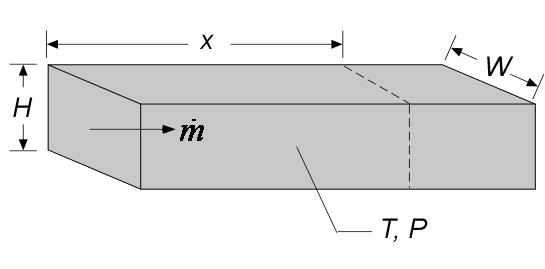Contents - Index

DuctFlow_localProcedure DuctFlow_local(Fluid\$, T, P, m_dot, H, W, x, RelRough: h_T_x, h_H_x,  dPdx)  returns lower and upper bounds on the local heat transfer coefficient and the local pressure gradient at axial position x for a specified mass flow rate (m_dot) through a rectangular duct of height H and width W.  The procedure assumes simultaneous hydrodynamic and thermally developing flow.  Properties are evaluated at the bulk temperature T and pressure P.  Units of the inputs and outputs depend upon the unit settings in EES.

Inputs:

Fluid\$ can be any fluid in the EES database.  The fluid can be an ideal gas or a real fluid.

T - the bulk temperature of the fluid at location x in  [C], [K], [F], or [R].

P -  pressure at location x in [Pa], [kPa], [bar], [MPa], [atm], or [psia].

m_dot  -  mass flow rate in [kg/s], or [lbm/hr]

H - duct height [m] or [ft]

W - duct width [m] or [ft]

x - position in the axial direction of the duct in [m] or [ft]

RelRough - the ratio of the dispersions on the wall of the duct to the duct hydraulic diameter (must be between 0 and 0.05)

Outputs:

h_T_x  -  local heat transfer coefficient in [W/m^2-K] or [Btu/hr-ft^2-R] assuming that the duct wall is at constant temperature (lower bound)

h_H_x  -  local heat transfer coefficient in [W/m^2-K] or [Btu/hr-ft^2-R] assuming a constant heat flux at the duct wall (upper bound)

dPdx -  local pressure gradient.  [(Pa, kPa, bar,  or atm)/m] or  [(psi or atm)/ft] depending on EES pressure unit setting

Notes

This procedure  differentiates the average heat transfer coefficient and pressure gradient  provided by procedure DuctFlow.

Two values of the local heat transfer coefficient are returned.  The first, h_T_x is determined assuming that the wall is at constant temperature.  The second (h_H_x) is determined assuming that the wall is subjected to a constant heat flux.  For laminar flow, these values should provide lower and upper bounds on the heat transfer coefficient as discussed in Section 5.2.4 of Nellis and Klein.   For turbulent flow, these values are identical.

Example:

\$unitSystem SI Pa K J

T=363 [K]  "average temperature of fluid in pipe at location x"

P=101.3 [Pa]  "pressure of air in pipe"

m_dot=0.05 [kg/s]  "flow rate"

x=5 [m]  "location along axial direction"

RelRough=0 [-] "relative roughness"

H=0.1 [m] "height"

W=0.2 [m]   "width"

Call ductflow_local( 'Air', T, P, m_dot, H,W, x,RelRough: h_T_x, h_H_x, dPdx)5

{Solution:

dPdx=-679.4 [Pa/m]

h_H_x=9.787 [W/m^2-K]

h_T_x=9.787 [W/m^2-K]}

Note that it is not necessary to evaluate all outputs for versions 9.513 or newer.  For example, if only h_H,x is of interest, the call statement can be:

Call ductflow_local( 'Air', T, P, m_dot, H,W, x,RelRough: , h_H_x, )

In the European system use the semicolon in place of the comma as the list separator.

Internal Flow Index